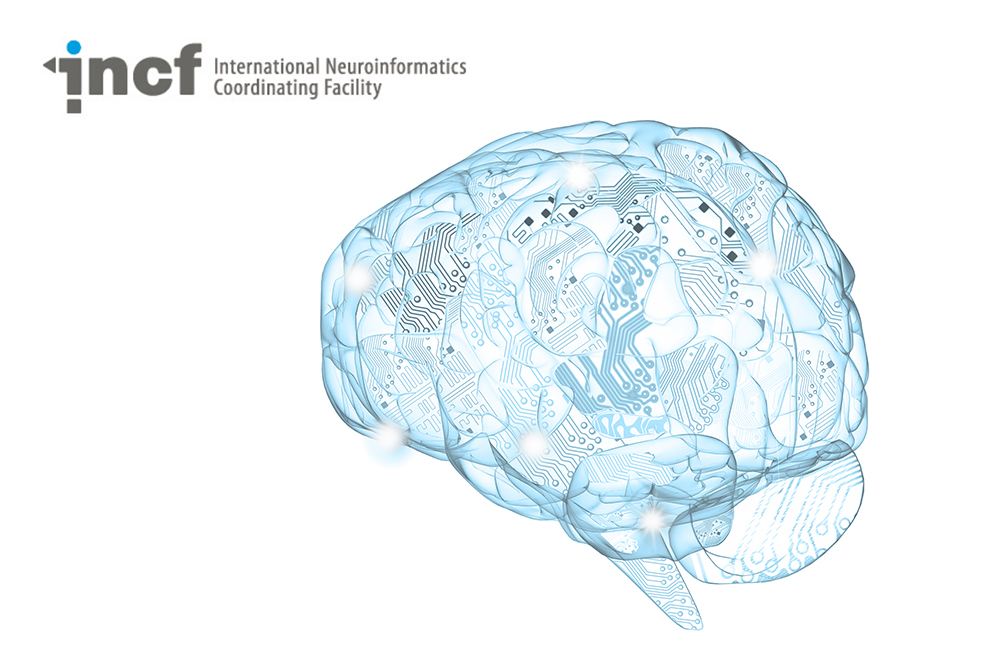# Module 4: fMRI

Category
Level
Intermediate

This module covers fMRI data, including creating and interpreting flatmaps, exploring variability and average responses, and visual eccenticity. You will learn about processing BOLD signals, trial-averaging, and t-tests. The MATLAB code introduces data animations, multicolor visualizations, and linear indexing.

Course Features
Tutorials
Data and code files
Exercises
Lessons of this Course
1
1
Duration:
7:15

This module covers fMRI data, including creating and interpreting flat maps, exploring variability and average responses, and visual eccentricity. You will learn about processing BOLD signals, trial-averaging, and t-tests. The MATLAB code introduces data animations, multicolor visualizations, and linear indexing.

2
2
Duration:
12:15

This module covers fMRI data, including creating and interpreting flatmaps, exploring variability and average responses, and visual eccentricity. You will learn about processing BOLD signals, trial-averaging, and t-tests. The MATLAB code introduces data animations, multicolor visualizations, and linear indexing.

3
3
Duration:
12:05

This module covers fMRI data, including creating and interpreting flatmaps, exploring variability and average responses, and visual eccentricity. You will learn about processing BOLD signals, trial-averaging, and t-tests. The MATLAB code introduces data animations, multicolor visualizations, and linear indexing.

4
4
Duration:
20:12

This module covers fMRI data, including creating and interpreting flatmaps, exploring variability and average responses, and visual eccentricity. You will learn about processing BOLD signals, trial-averaging, and t-tests. The MATLAB code introduces data animations, multicolor visualizations, and linear indexing.

5
5
Duration:
12:52

This module covers fMRI data, including creating and interpreting flatmaps, exploring variability and average responses, and visual eccentricity. You will learn about processing BOLD signals, trial-averaging, and t-tests. The MATLAB code introduces data animations, multicolor visualizations, and linear indexing.

6
6
Duration:
13:39

This module covers fMRI data, including creating and interpreting flatmaps, exploring variability and average responses, and visual eccentricity. You will learn about processing BOLD signals, trial-averaging, and t-tests. The MATLAB code introduces data animations, multicolor visualizations, and linear indexing.

7
7
Duration:
17:54

This module covers fMRI data, including creating and interpreting flatmaps, exploring variability and average responses, and visual eccentricity. You will learn about processing BOLD signals, trial-averaging, and t-tests. The MATLAB code introduces data animations, multicolor visualizations, and linear indexing.

##### Recent courses#### Introduction to deep learning#### Cellular Mechanisms of Brain Function